Next: Jacobian construction Up: Iterative methods Previous: Iterative methods

### Jacobian inversion method

The Jacobian is the multidimensional extension to the differentiation of a single variable [WW92]. As it was mentioned in the introduction, there is a relation between the Cartesian space of the end effector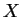and the joint space of the joint angles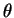. The Jacobian, in fact, transforms the differential angle changes to the differential motions of the end effector [Mck91].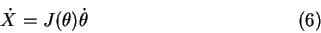The vector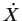represents the linear velocity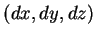and rotational velocity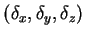of the end effector and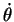represents time derivative of the state vector (rotational velocity for each joint).

Since the unknown is, the Jacobian inversion is needed. Hence, the equation (6) is transformed to the form: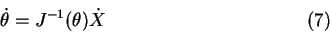Lukas Barinka 2002-03-21